Freestyle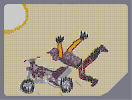Hover over the thumbnail for a full-size version.

Author ghazbaran author:ghazbaran n-art nonplayable rated 2007-10-23 3 by 14 people. \$Freestyle#ghazbaran#none#9000000000000000000000600000000000000000000000000000000000000000000000000000000000000000000000000000000000000000000000000000000000000000000000000000000000000000000000000000000000000000000000000000000000000000000000000000000000000000000000000000000000000000000000000000000000000000000000000000000000000000000000000000000000000000000000000000000000000000000000000000000000000000000000000000000000000000000000000000000000000000000000000000000000000000000000000000000000000000000000000000000000000000000000000000000000000000000000000000000000000000000000000000000000000000000000?0000000000000000000000C0000000000000000000003100000000000000000000311000000000000000000031110000000000000000003111180000000000000000311111|5^492,204!5^516,223!5^526,215!2^180,414,-1,0!2^180,432,-1,0!2^174,438,-1,0!2^174,444,-1,0!2^174,450,-1,0!2^174,462,-1,0!2^168,462,-1,0!2^168,468,-1,0!2^168,468,-1,0!2^168,474,-1,0!2^174,474,-1,0!2^174,468,-1,0!2^174,468,-1,0!2^174,462,-1,0!2^180,462,-1,0!2^180,462,-1,0!2^180,456,-1,0!2^180,450,-1,0!2^180,444,-1,0!2^180,438,-1,0!2^180,438,-1,0!2^180,432,-1,0!2^186,438,-1,0!2^186,432,-1,0!2^186,426,-1,0!2^186,426,-1,0!2^186,414,-1,0!2^168,486,-1,0!2^168,486,-1,0!2^168,492,-1,0!2^168,498,-1,0!2^168,504,-1,0!2^168,504,-1,0!2^168,504,-1,0!2^162,498,0.707106781186547,0.707106781186547!2^156,498,0.707106781186547,0.707106781186547!2^156,504,0.707106781186547,0.707106781186547!2^156,510,1,0!2^162,516,0.707106781186547,-0.707106781186547!2^168,516,0.707106781186547,-0.707106781186547!2^174,516,-0.707106781186547,-0.707106781186547!2^174,510,-0.707106781186547,-0.707106781186547!2^174,504,-0.707106781186547,0.707106781186547!2^174,498,-0.707106781186547,0.707106781186547!2^156,492,0.707106781186547,-0.707106781186547!2^144,504,0,1!2^132,504,0,1!2^156,516,0.707106781186547,0.707106781186547!2^144,528,0.707106781186547,0.707106781186547!2^168,516,0,1!2^168,516,1,0!2^168,528,1,0!2^168,540,1,0!2^180,516,0.707106781186547,-0.707106781186547!2^192,528,0.707106781186547,-0.707106781186547!2^180,504,0.707106781186547,0.707106781186547!2^192,492,0.707106781186547,0.707106781186547!2^168,492,1,0!2^168,492,0.707106781186547,0.707106781186547!2^180,480,0.707106781186547,0.707106781186547!5^174,552!5^184,550!5^192,548!5^194,543!5^198,539!5^199,537!5^200,530!5^201,525!5^203,520!5^204,515!5^204,511!5^204,507!5^204,506!5^204,501!5^204,495!5^202,492!5^196,486!5^187,480!5^181,480!5^180,480!5^125,517!5^127,524!5^134,531!5^140,535!5^154,548!5^168,553!5^154,549!2^180,402,1,0!2^180,390,1,0!2^180,378,1,0!2^186,414,1,0!2^186,402,1,0!2^186,402,1,0!2^186,390,1,0!2^186,378,1,0!3^162,419!3^166,418!3^173,418!3^193,430!3^178,422!3^192,425!3^196,435!3^201,435!3^206,438!3^208,441!3^213,447!2^210,462,1,0!2^210,474,1,0!2^204,486,0.707106781186547,0.707106781186547!2^210,492,0,1!2^210,486,0.707106781186547,0.707106781186547!2^216,480,1,0!2^216,468,1,0!2^216,462,1,0!5^196,528!5^194,520!5^194,506!5^192,502!5^176,485!5^165,484!5^170,485!5^191,492!5^186,492!5^160,487!5^159,487!5^154,488!5^150,488!5^134,499!5^132,504!5^132,513!5^140,496!5^143,492!5^147,489!5^138,518!5^147,525!5^150,526!5^155,531!5^163,537!5^172,537!5^190,537!5^174,543!5^161,545!5^182,540!5^190,531!5^195,524!5^195,511!5^136,523!5^158,550!3^221,452!3^231,453!3^234,454!3^238,454!3^246,456!3^246,456!3^251,460!3^251,460!3^254,467!3^254,469!2^222,480,0.707106781186547,0.707106781186547!2^228,480,0.707106781186547,0.707106781186547!2^234,480,0.707106781186547,0.707106781186547!2^240,480,0.707106781186547,0.707106781186547!2^246,480,0.707106781186547,0.707106781186547!2^252,486,0.707106781186547,0.707106781186547!2^258,486,0.707106781186547,0.707106781186547!2^258,486,0.707106781186547,0.707106781186547!2^252,480,0.707106781186547,0.707106781186547!2^252,480,0.707106781186547,0.707106781186547!2^270,480,0.707106781186547,0.707106781186547!2^270,480,0.707106781186547,0.707106781186547!2^270,480,0.707106781186547,0.707106781186547!2^276,480,0.707106781186547,0.707106781186547!2^282,486,-1,0!2^282,486,-1,0!1^276,486!2^282,474,0.707106781186547,0.707106781186547!2^282,468,0.707106781186547,0.707106781186547!2^282,462,0.707106781186547,0.707106781186547!2^282,456,0.707106781186547,0.707106781186547!2^282,456,0.707106781186547,0.707106781186547!2^282,450,0.707106781186547,0.707106781186547!2^282,450,0.707106781186547,0.707106781186547!2^216,492,0.707106781186547,0.707106781186547!2^222,492,0.707106781186547,0.707106781186547!2^228,492,0.707106781186547,0.707106781186547!2^240,492,0.707106781186547,0.707106781186547!2^246,492,0.707106781186547,0.707106781186547!2^252,492,0.707106781186547,0.707106781186547!2^252,492,0.707106781186547,0.707106781186547!2^258,492,0.707106781186547,0.707106781186547!2^270,492,0.707106781186547,0.707106781186547!2^276,492,0.707106781186547,0.707106781186547!1^276,486!3^257,456!3^266,453!3^273,447!3^279,445!3^284,445!3^293,445!3^303,449!3^309,451!3^318,458!10^335,436!10^332,440!10^330,449!10^329,457!10^330,459!10^340,461!10^343,461!10^344,460!10^349,454!10^353,450!10^357,445!10^360,439!10^357,439!10^353,437!10^345,430!10^343,429!10^339,437!10^341,454!10^334,456!10^345,444!10^350,442!10^346,444!10^339,445!10^324,450!10^342,444!10^348,444!10^348,432!10^354,438!10^336,468!10^324,456!10^318,456!10^330,462!10^324,462!10^330,468!10^336,474!10^342,468!10^348,462!10^354,456!10^360,450!10^366,444!10^360,438!10^354,432!10^348,426!10^342,432!10^330,444!10^361,433!10^365,426!10^368,423!10^371,421!10^373,418!10^377,415!10^385,410!10^390,406!10^391,406!10^380,409!10^367,440!10^378,432!10^384,426!10^390,420!10^396,414!10^402,408!10^408,402!10^402,408!10^402,402!10^384,420!10^378,426!10^366,432!10^360,432!10^360,432!10^366,438!10^372,438!10^372,432!10^378,426!10^378,420!10^384,420!10^390,414!10^390,414!10^384,414!10^378,426!10^366,438!10^336,450!10^336,450!10^342,450!10^342,456!3^288,480!3^294,472!3^297,476!3^301,472!3^303,469!3^306,467!3^312,465!3^319,461!10^299,484!10^303,484!10^306,484!10^312,487!10^315,489!10^321,492!10^325,494!10^332,501!10^342,508!10^349,512!10^356,514!10^351,513!10^343,508!10^339,505!10^333,499!10^299,488!10^302,488!10^309,492!10^320,499!10^326,503!10^330,506!10^338,510!10^341,511!3^342,476!3^342,485!3^342,497!3^344,498!3^342,484!3^335,475!3^336,486!3^334,493!1^363,500!1^362,497!1^361,492!1^359,482!1^360,474!2^366,462,0.707106781186547,0.707106781186547!2^372,450,0.707106781186547,0.707106781186547!2^384,444,0.707106781186547,0.707106781186547!2^384,444,0.707106781186547,0.707106781186547!2^390,438,0.707106781186547,0.707106781186547!2^402,432,0.707106781186547,0.707106781186547!2^402,432,0.707106781186547,0.707106781186547!2^402,432,0.707106781186547,0.707106781186547!2^390,438,0.707106781186547,0.707106781186547!2^384,456,0.707106781186547,0.707106781186547!2^378,456,0.707106781186547,0.707106781186547!2^372,462,0.707106781186547,0.707106781186547!2^372,468,0.707106781186547,0.707106781186547!2^372,468,0.707106781186547,0.707106781186547!2^384,462,0.707106781186547,0.707106781186547!2^390,456,0.707106781186547,0.707106781186547!2^396,456,0.707106781186547,0.707106781186547!2^402,444,0.707106781186547,0.707106781186547!2^408,444,0.707106781186547,0.707106781186547!2^408,438,0.707106781186547,0.707106781186547!2^414,438,0.707106781186547,0.707106781186547!2^420,432,0.707106781186547,0.707106781186547!2^420,432,0.707106781186547,0.707106781186547!2^420,426,0.707106781186547,0.707106781186547!2^420,426,0.707106781186547,0.707106781186547!2^420,420,0.707106781186547,0.707106781186547!2^420,414,0.707106781186547,0.707106781186547!2^408,420,0.707106781186547,0.707106781186547!2^402,426,0.707106781186547,0.707106781186547!2^384,438,0.707106781186547,0.707106781186547!2^396,432,0.707106781186547,0.707106781186547!2^396,426,0.707106781186547,0.707106781186547!2^408,414,0.707106781186547,0.707106781186547!2^414,414,0.707106781186547,0.707106781186547!2^408,426,0.707106781186547,0.707106781186547!2^414,426,0.707106781186547,0.707106781186547!2^408,432,0.707106781186547,0.707106781186547!2^402,438,0.707106781186547,0.707106781186547!2^402,438,0.707106781186547,0.707106781186547!2^396,444,0.707106781186547,0.707106781186547!2^390,450,0.707106781186547,0.707106781186547!2^384,438,0.707106781186547,0.707106781186547!2^384,438,0.707106781186547,0.707106781186547!2^366,462,0.707106781186547,0.707106781186547!2^360,462,0.707106781186547,0.707106781186547!2^312,474,0,1!2^324,474,0,1!2^336,480,0,1!2^348,480,0,1!2^360,480,0,1!2^372,486,0,1!2^378,492,1,0!2^378,510,1,0!2^378,504,1,0!2^378,522,0,1!2^366,522,0,1!2^360,522,0,1!2^348,522,0,1!2^378,522,1,0!2^378,522,0.707106781186547,0.707106781186547!2^378,486,0.707106781186547,-0.707106781186547!2^372,486,0,-1!2^336,522,-0.707106781186547,0.707106781186547!2^324,516,-0.707106781186547,0.707106781186547!2^312,510,-0.707106781186547,0.707106781186547!2^300,504,-0.707106781186547,0.707106781186547!2^294,498,-0.707106781186547,0.707106781186547!5^365,516!5^365,512!5^365,505!5^368,497!5^368,495!5^370,493!5^381,477!5^393,472!5^396,470!5^396,470!5^412,473!5^416,477!5^420,486!5^421,491!5^421,498!5^421,507!5^421,515!5^414,529!5^400,536!5^394,540!5^391,540!5^387,541!5^384,543!5^378,538!5^375,529!5^373,520!5^384,534!5^398,539!5^414,533!5^420,520!5^427,506!5^427,492!5^420,481!5^413,476!5^404,475!5^395,475!5^370,480!5^365,500!5^365,514!5^377,547!5^385,546!5^394,546!5^406,546!5^417,545!5^423,535!5^434,525!5^436,513!5^436,504!5^427,481!5^420,472!5^398,467!5^395,468!5^384,481!5^365,509!5^367,516!5^367,522!5^367,531!5^374,543!5^388,546!5^395,546!5^414,541!5^423,536!5^429,520!5^431,506!5^432,492!5^429,477!5^431,491!5^433,501!5^431,511!5^429,522!5^417,539!5^382,484!5^387,479!1^182,366!1^188,362!1^190,362!1^192,362!1^197,362!1^201,361!2^209,353,0.707106781186547,0.707106781186547!2^216,347,0.707106781186547,0.707106781186547!2^173,359,0,1!2^161,359,0,1!12^226,343!12^228,343!12^232,343!12^235,343!12^238,343!12^152,363!12^152,363!12^149,367!12^149,367!12^145,371!2^396,492,0.707106781186547,0.707106781186547!2^396,492,0.707106781186547,0.707106781186547!2^396,498,0.707106781186547,0.707106781186547!2^396,498,0.707106781186547,0.707106781186547!2^396,504,0.707106781186547,0.707106781186547!2^396,522,0.707106781186547,0.707106781186547!2^396,522,0.707106781186547,0.707106781186547!2^402,516,0.707106781186547,0.707106781186547!2^402,510,0.707106781186547,0.707106781186547!2^402,510,0.707106781186547,0.707106781186547!2^396,510,0.707106781186547,0.707106781186547!2^396,510,0.707106781186547,0.707106781186547!2^396,516,0.707106781186547,0.707106781186547!2^372,510,0,1!2^378,510,0,1!2^384,510,0,1!2^384,510,0,1!2^396,510,0,1!2^402,510,0,1!2^408,510,0,1!2^414,510,0,1!2^414,510,0,1!2^414,510,0,1!2^384,492,0.707106781186547,0.707106781186547!2^390,504,0.707106781186547,0.707106781186547!2^396,510,0.707106781186547,0.707106781186547!2^408,498,0.707106781186547,-0.707106781186547!2^408,492,0.707106781186547,-0.707106781186547!5^372,510!5^366,510!5^366,522!10^161,410!10^164,407!10^174,407!10^177,407!10^181,409!10^192,417!10^197,422!10^198,424!10^202,426!10^207,429!10^213,432!10^220,434!10^179,415!10^187,414!10^189,418!10^204,434!10^211,419!10^214,417!10^224,412!10^229,409!10^221,434!10^226,429!10^230,416!10^234,412!10^221,418!10^212,423!10^222,426!10^226,423!10^230,417!10^238,417!10^221,436!10^221,436!10^228,426!10^236,427!10^246,429!10^250,432!10^259,435!10^255,435!10^245,435!10^240,435!10^230,432!10^229,432!10^211,434!10^220,443!10^220,443!10^241,447!10^248,449!10^253,451!10^259,451!10^261,449!10^256,449!10^244,449!10^232,445!10^222,438!10^249,440!10^254,440!10^260,440!10^242,466!10^242,466!10^232,458!10^229,456!10^234,459!10^193,439!10^200,443!10^206,449!10^206,452!10^206,456!10^206,459!10^206,466!10^197,453!10^197,449!10^195,445!10^199,460!10^378,406!10^378,406!10^376,406!10^375,406!10^373,406!10^370,407!10^367,407!10^363,409!10^357,411!10^350,414!10^347,418!10^344,420!10^336,423!10^333,423!10^323,423!10^322,423!10^312,420!10^312,420!10^288,413!10^288,413!10^296,415!10^301,415!10^304,415!10^311,417!1^290,416!1^295,419!1^301,419!1^307,420!1^311,421!1^321,424!1^328,426!10^337,428!10^335,429!10^331,430!10^325,430!10^320,430!10^317,430!10^311,428!10^308,426!10^303,425!10^296,422!10^293,422!10^292,422!10^287,420!10^284,418!10^280,413!10^274,413!10^272,413!10^268,411!10^263,407!10^256,405!10^253,403!10^253,403!10^252,403!10^251,403!10^249,403!10^233,402!10^232,402!10^240,402!10^246,402!10^242,402!2^271,425,0,1!2^271,425,0,1!2^276,425,0,1!2^284,430,0,1!2^292,432,0,1!2^299,433,0,1!2^302,434,0,1!2^272,433,0.707106781186547,-0.707106781186547!2^278,433,0.707106781186547,-0.707106781186547!2^284,434,0.707106781186547,-0.707106781186547!2^285,434,0.707106781186547,-0.707106781186547!2^292,434,0.707106781186547,-0.707106781186547!2^299,435,0.707106781186547,-0.707106781186547!10^321,441!10^317,445!10^314,446!10^323,438!10^318,438!10^270,436!10^267,434!10^256,425!10^246,418!10^246,415!10^247,413!10^271,417!10^273,418!10^266,419!10^255,416!10^253,413!10^254,413!10^226,398!10^224,398!10^219,394!10^217,392!10^214,389!10^205,383!10^205,382!10^203,378!8^200,362,1!8^193,364,1!8^191,364,1!8^189,366,1!8^186,368,1!10^206,374!10^202,374!10^195,375!10^193,377!10^189,378!10^186,378!10^183,378!10^204,372!10^196,385!10^195,386!10^196,386!10^196,387!10^196,387!10^196,390!10^196,392!10^196,395!10^196,400!10^196,407!10^196,412!2^207,418,0.707106781186547,0.707106781186547!2^211,412,0.707106781186547,0.707106781186547!2^216,408,0.707106781186547,0.707106781186547!2^219,406,0.707106781186547,0.707106781186547!2^221,404,0.707106781186547,0.707106781186547!2^206,394,0.707106781186547,-0.707106781186547!2^209,398,0.707106781186547,-0.707106781186547!2^216,404,0.707106781186547,-0.707106781186547!2^217,404,0.707106781186547,-0.707106781186547!2^205,416,-1,0!2^205,405,-1,0!2^204,401,-1,0!2^204,395,-1,0!2^208,402,0.707106781186547,0.707106781186547!2^210,406,0.707106781186547,0.707106781186547!2^206,399,0.707106781186547,0.707106781186547!12^282,317!12^282,317!12^290,315!12^294,313!12^303,307!12^305,305!12^300,309!12^311,300!12^312,299!12^317,293!12^319,291!12^324,289!12^328,289!12^332,289!12^346,319!12^346,328!12^343,331!12^347,325!12^340,335!12^339,335!12^337,336!12^335,342!12^335,347!12^337,354!12^347,335!12^347,345!12^344,351!12^342,355!12^340,359!12^338,361!12^335,361!12^332,361!12^331,361!12^349,339!12^327,358!12^324,358!12^323,356!12^320,355!12^318,354!12^319,349!12^320,339!12^321,336!12^324,333!12^331,327!12^335,323!12^317,344!12^326,328!12^283,316!12^290,318!12^295,317!12^300,317!12^303,317!12^303,317!12^307,317!12^310,317!12^320,317!12^323,317!12^326,316!12^331,312!12^334,306!12^315,319!12^333,306!12^333,309!12^339,310!12^342,315!12^344,315!12^336,290!12^336,290!12^337,290!12^337,290!12^341,294!12^338,320!9^312,325,1,0,14,0,1,0,0!9^317,329,1,0,14,0,1,0,0!9^320,324,1,0,14,0,1,0,0!9^326,322,1,0,14,0,1,0,0!9^333,319,1,0,14,0,1,0,0!9^336,315,1,0,14,0,1,0,0!9^304,325,1,0,14,0,1,0,0!9^309,333,1,0,14,0,1,0,0!9^314,336,1,0,14,0,1,0,0!0^355,335!0^355,333!0^355,327!0^355,323!0^353,314!0^353,305!0^349,298!0^348,287!0^348,274!0^348,274!0^350,290!0^348,292!0^348,280!0^354,318!0^354,312!0^354,306!0^356,337!0^360,339!0^363,339!0^367,339!0^371,334!0^371,322!0^346,273!0^346,270!0^347,266!0^347,262!0^347,261!0^368,314!0^368,314!0^368,309!0^376,313!0^375,308!0^375,306!0^373,303!0^373,298!0^373,298!0^371,290!0^370,287!0^369,284!0^367,277!0^366,274!0^365,270!0^365,263!0^365,259!0^374,294!12^348,257!12^355,257!12^359,257!12^362,257!12^351,257!12^366,257!5^346,250!5^345,248!5^340,243!5^340,243!5^338,234!5^337,235!5^342,239!5^348,235!5^349,234!5^349,227!5^350,223!5^354,231!5^356,229!5^361,235!5^364,232!5^364,229!5^364,227!5^364,221!5^364,221!5^367,236!5^370,236!5^373,235!5^377,232!5^380,229!5^382,227!5^375,239!5^370,244!5^365,248!5^365,252!5^363,250!5^357,250!5^355,248!5^352,245!5^358,245!12^342,306!12^342,300!12^348,312!12^348,306!12^348,318!12^348,324!11^342,6,306,311!11^348,6,316,312!11^343,4,317,306!11^346,4,326,307!11^352,4,322,300!11^345,2,334,300!11^347,10,328,295!11^356,13,340,345!11^350,11,328,351!11^349,4,327,345!11^356,4,330,338!11^352,6,335,330!11^359,9,341,325!12^337,324!12^330,329!12^347,333!12^344,321!12^347,328!12^340,339!12^347,339!12^335,342!12^335,344!12^336,346!12^336,347!12^338,353!12^344,343!12^340,345!12^339,349!12^341,352!9^345,347,1,0,17,0,1,0,0!9^343,351,1,0,17,0,1,0,0!9^343,344,1,0,18,0,1,0,0!9^347,343,1,0,18,0,1,0,0!0^366,330!0^366,336!0^360,336!0^360,330!0^360,324!0^366,324!0^366,330!0^372,312!0^372,306!0^372,300!0^372,294!0^372,288!0^372,312!0^366,324!0^360,318!0^360,306!0^360,306!0^360,300!0^360,294!0^354,294!0^354,288!0^354,276!0^354,264!0^360,264!0^360,270!0^360,276!0^366,282!0^366,294!0^366,294!0^366,312!0^360,318!0^360,312!0^366,318!0^366,300!0^366,300!0^354,300!0^366,306!0^366,282!0^366,288!0^360,288!0^360,282!0^354,282!0^348,276!0^348,282!0^348,288!0^354,270!0^360,270!0^348,270!0^360,264!0^354,264!12^366,258!12^360,258!12^354,258!12^354,258!12^348,258!0^372,332!0^372,328!0^372,324!0^372,320!0^372,338!10^355,345!10^360,348!10^368,348!10^372,348!10^377,348!3^381,337!3^382,336!3^388,331!3^392,328!3^394,326!3^397,322!3^403,320!3^405,317!3^409,314!3^385,342!3^399,349!3^399,349!3^394,346!3^406,353!3^406,353!3^417,361!3^418,361!3^422,362!3^427,365!3^431,365!3^437,368!3^443,371!3^453,376!3^457,379!3^457,379!3^465,379!3^467,380!3^470,382!3^479,364!3^475,371!3^481,355!10^351,357!10^360,359!10^368,363!10^370,366!10^375,371!10^385,377!10^386,379!10^392,381!10^398,383!10^402,384!10^408,389!10^418,393!10^422,393!10^427,393!10^433,394!10^437,397!10^445,401!10^448,402!10^451,402!10^454,401!10^462,392!10^462,390!10^450,396!10^450,396!10^450,390!10^444,384!10^438,378!10^432,372!10^420,372!10^414,366!10^408,360!10^396,354!10^384,348!10^384,354!10^372,354!10^366,354!10^378,360!10^378,366!10^384,372!10^390,372!10^396,378!10^408,384!10^414,390!10^426,390!10^438,390!10^444,390!10^426,384!10^426,384!10^420,378!10^408,372!10^402,372!10^390,366!10^384,360!10^384,360!10^402,366!10^408,366!10^390,360!10^396,360!10^408,366!10^426,384!10^432,384!10^432,384!10^426,378!10^414,378!10^408,378!10^402,366!10^360,348!10^360,348!10^360,354!10^378,330!10^390,318!10^384,324!10^396,312!10^402,306!3^419,312!3^428,318!3^432,322!3^434,324!3^438,328!3^442,332!3^444,334!3^450,336!3^460,343!3^464,345!3^468,347!3^470,348!3^475,352!3^415,312!3^390,336!3^390,336!3^408,342!3^408,342!3^426,354!3^444,354!3^456,360!3^462,372!3^468,366!3^468,360!3^468,360!3^468,360!3^462,360!3^456,366!3^444,366!3^444,360!3^462,360!3^468,360!3^456,354!3^456,354!3^432,354!3^432,354!3^444,354!3^444,348!3^450,342!3^450,342!3^450,342!3^456,348!3^444,348!3^420,354!3^420,348!3^414,348!3^414,348!3^408,336!3^402,336!3^396,336!3^408,330!3^414,324!3^420,324!3^408,330!3^420,324!3^420,318!3^420,330!3^420,336!3^426,336!3^438,342!3^444,342!3^426,336!3^426,348!3^426,342!3^426,330!3^426,330!3^432,330!3^438,342!5^461,405!5^464,402!5^471,394!5^473,390!5^478,385!5^481,381!5^486,376!5^491,370!5^491,364!5^491,355!5^487,367!5^487,376!5^476,388!5^466,396!5^493,373!5^486,376!12^472,412!12^472,413!12^473,420!12^474,426!12^476,435!12^476,438!12^476,448!12^475,430!12^477,444!12^477,453!12^477,454!12^478,456!12^479,461!12^479,464!12^483,468!12^487,471!12^489,472!12^495,473!12^496,475!12^499,477!12^506,479!12^507,479!12^507,407!12^507,407!12^507,412!12^507,416!12^507,426!12^507,429!12^507,436!12^507,443!12^507,445!12^507,423!12^507,447!12^510,449!12^521,457!12^521,457!12^515,454!12^515,480!12^513,480!12^509,406!12^509,406!12^514,403!12^520,398!12^523,395!12^523,394!12^529,389!12^531,387!12^538,382!12^541,380!12^535,382!12^535,383!12^549,374!12^551,372!12^551,370!12^553,368!12^546,377!12^502,368!12^506,364!12^510,361!12^514,356!12^519,350!12^525,346!12^530,342!12^531,339!12^531,339!12^521,350!10^520,482!10^523,477!10^526,470!10^528,460!10^526,458!10^526,462!10^522,474!10^532,336!10^537,339!10^542,342!10^543,344!10^551,353!10^552,356!10^556,362!10^557,363!10^547,351!10^541,345!10^539,339!10^550,348!10^530,466!10^527,466!5^483,454!5^483,454!5^488,454!5^491,454!5^493,454!5^496,454!5^500,454!5^507,454!5^507,458!5^507,459!5^504,466!5^501,470!5^488,392!5^489,394!5^490,398!5^491,408!5^491,415!5^492,422!5^493,430!5^493,437!5^493,442!5^498,397!5^502,390!5^503,387!5^504,379!5^504,373!5^509,383!5^511,383!5^522,373!5^524,370!5^518,379!5^527,369!5^530,365!5^538,355!5^538,355!5^538,357!12^532,352!12^529,353!12^525,358!12^522,360!12^520,362!12^518,365!12^511,370!12^507,373!12^505,372!12^508,366!12^515,362!12^525,356!12^513,369!12^518,354!12^530,347!12^535,357!12^528,360!12^519,365!12^545,362!12^545,364!12^534,371!12^527,375!12^523,380!12^518,386!12^511,392!12^514,390!12^519,389!12^525,385!12^533,381!12^538,378!12^543,370!12^543,367!12^535,371!12^535,372!12^542,368!12^530,375!12^517,391!12^513,397!12^509,400!5^511,382!5^511,377!5^515,374!5^518,371!5^523,370!5^531,367!5^533,363!5^490,407!5^489,414!5^492,421!5^493,428!5^493,435!5^493,440!12^502,442!12^502,437!12^503,432!12^502,426!12^502,424!12^502,421!12^502,413!12^500,409!12^502,405!12^506,397!12^480,405!12^484,401!12^498,384!12^496,384!12^502,377!12^509,374!12^475,409!12^474,407!12^479,415!12^480,420!12^480,443!12^486,443!12^483,432!12^482,426!12^483,413!12^483,410!12^480,421!12^478,431!12^480,435!12^481,435!12^477,417!12^480,426!12^479,432!12^491,382!12^494,466!12^489,466!12^487,466!12^510,473!12^514,472!12^515,468!12^515,466!12^517,464!12^518,459!12^519,457!12^540,360!12^508,471!5^512,468!5^515,469!5^516,470!5^505,462!5^509,465!5^537,463!5^541,464!5^545,466!5^551,469!5^555,471!5^550,479!5^549,478!5^546,478!5^536,478!5^532,478!5^534,474!5^531,482!5^549,487!5^552,487!5^540,478!5^547,484!5^543,482!5^531,476!10^560,477!10^560,479!10^560,484!10^560,494!10^560,496!10^559,501!10^557,505!10^552,510!10^551,512!10^551,515!10^556,515!10^557,515!10^560,514!10^563,512!10^566,509!10^570,506!10^573,503!10^577,498!10^580,496!10^580,492!10^581,490!10^582,488!10^582,487!10^586,482!10^581,480!10^572,477!10^569,476!10^565,476!10^571,479!10^573,484!10^572,488!10^568,495!10^566,501!10^566,505!10^570,494!10^570,482!10^568,482!10^568,490!5^563,354!5^563,354!5^567,351!5^570,348!5^576,343!5^580,337!5^583,333!5^585,329!5^587,323!5^590,318!5^590,315!5^545,329!5^545,329!5^553,326!5^556,316!5^552,322!5^551,324!5^567,310!5^565,310!5^564,310!5^566,307!5^567,306!5^571,303!5^574,301!5^580,296!5^586,292!5^587,292!5^593,298!5^596,299!5^598,299!5^599,301!5^600,305!5^600,306!5^598,312!5^593,316!5^559,346!5^559,341!5^555,336!5^554,334!5^566,335!5^572,339!5^569,337!5^570,332!5^575,329!5^579,324!5^583,319!5^588,311!5^583,314!5^573,319!5^561,327!5^549,333!5^549,330!5^555,324!5^568,317!5^577,309!5^582,304!5^581,300!10^593,290!10^597,286!10^601,284!10^603,281!10^605,279!10^606,277!10^609,272!10^609,272!10^614,274!10^619,277!10^628,285!10^628,286!10^630,292!10^635,295!10^639,300!10^639,300!10^642,303!10^645,306!10^645,307!10^611,308!10^612,306!10^615,303!10^617,301!10^617,301!10^619,301!10^621,304!10^622,305!10^625,308!10^633,309!10^641,309!10^636,304!10^632,300!10^630,298!10^627,296!10^625,293!10^622,290!10^621,289!10^614,283!10^614,286!10^611,294!10^608,297!10^606,294!10^605,292!10^608,286!0^406,302!0^416,307!0^418,307!0^427,310!0^430,311!0^419,307!0^414,303!0^408,299!0^416,290!0^420,286!0^424,285!0^410,297!0^410,295!0^421,281!0^420,279!0^420,277!0^424,275!0^428,274!0^433,268!0^438,263!0^441,261!0^445,256!0^449,252!0^453,247!0^431,304!0^432,303!0^437,298!0^441,294!0^443,291!0^444,289!0^445,288!0^454,281!0^455,279!0^457,277!0^461,273!0^465,270!0^466,269!0^469,265!0^470,263!0^451,281!0^454,283!0^473,259!0^480,254!0^484,251!0^488,246!0^491,240!0^459,242!0^460,242!0^466,238!0^467,235!0^472,234!0^477,254!12^478,232!12^480,232!12^484,233!12^487,234!12^492,239!12^493,239!5^482,225!5^482,225!5^485,222!5^488,218!5^489,216!5^490,213!5^490,210!5^490,205!5^490,195!5^499,211!5^499,211!5^505,205!5^507,202!5^511,198!5^506,208!5^506,211!5^513,211!5^523,212!5^525,213!5^513,213!5^503,213!5^505,215!5^514,220!5^518,224!5^505,221!5^501,225!5^491,233!5^493,232!5^503,227!5^504,229!5^502,233!5^496,227!5^495,227!5^491,223!5^484,223!5^486,224!5^487,197!5^507,202!0^426,306!0^426,306!0^420,300!0^420,300!0^414,294!0^420,294!0^420,294!0^426,288!0^426,282!0^426,282!0^426,276!0^432,276!0^432,276!0^438,270!0^438,264!0^444,264!0^450,258!0^456,252!0^456,252!0^462,246!0^468,240!0^468,234!0^480,234!0^480,234!0^486,240!0^486,240!0^486,240!0^480,240!0^480,240!0^480,240!0^480,246!0^480,246!0^474,240!0^474,246!0^474,246!0^474,252!0^468,258!0^456,270!0^444,282!0^432,294!0^432,300!0^426,300!0^426,300!0^426,300!0^426,294!0^426,294!0^438,288!0^438,288!0^432,282!0^432,282!0^432,282!0^432,282!0^432,288!0^438,282!0^438,276!0^450,276!0^444,276!0^438,294!0^420,288!0^420,282!0^414,300!0^462,270!0^468,264!0^468,258!0^468,258!0^474,258!0^468,252!0^468,246!0^468,246!0^462,246!0^462,246!0^462,252!0^462,258!0^462,264!0^450,264!0^456,264!0^456,258!0^450,258!0^456,252!0^450,276!0^450,270!0^444,270!0^456,246!0^450,282!12^490,238!12^489,238!12^488,236!12^486,235!12^485,235!12^484,235!12^481,233!0^68,194!0^77,191!0^82,189!0^92,181!0^92,181!0^102,177!0^109,173!0^115,171!0^121,166!0^129,161!0^132,156!0^144,146!0^149,141!0^152,138!0^157,130!0^164,116!0^166,106!0^166,99!0^166,89!0^166,78!0^165,67!0^164,58!0^161,52!0^155,37!0^153,34!0^149,26!0^56,199!0^52,199!0^46,199!0^42,196!0^34,192!0^33,191!0^136,148!0^158,122!0^150,126!0^150,132!0^144,144!0^132,150!0^120,162!0^114,162!0^102,162!0^96,174!0^84,180!0^72,180!0^48,180!0^42,186!0^30,180!0^36,180!0^60,186!0^60,192!0^60,192!0^48,186!0^48,192!0^48,192!0^90,180!0^78,180!0^78,180!0^72,186!0^90,174!0^90,168!0^102,168!0^108,168!0^114,168!0^126,156!0^126,156!0^126,156!0^120,156!0^120,156!0^114,156!0^138,150!0^138,144!0^144,138!0^144,132!0^132,564!0^120,564!0^108,564!0^96,564!0^84,564!0^72,564!0^60,564!0^36,564!0^48,564!0^36,576!0^48,576!0^60,576!0^72,576!0^96,576!0^84,576!0^108,576!0^132,576!0^132,576!0^120,576!0^156,576!0^144,576!0^168,576!0^180,576!0^192,576!0^144,564!0^204,564!0^204,576!0^216,552!0^216,540!0^228,528!0^228,516!0^240,516!0^216,504!0^216,516!0^228,504!0^240,504!0^252,504!0^264,504!0^276,504!0^288,504!0^300,516!0^312,528!0^324,528!0^348,540!0^360,540!0^360,528!0^348,528!0^336,528!0^336,540!0^336,540!0^324,540!0^312,540!0^300,528!0^288,516!0^288,516!0^264,516!0^276,516!0^252,516!0^240,528!0^216,528!0^204,552!0^192,564!0^180,564!0^168,564!0^156,564!0^144,552!0^132,552!0^120,552!0^108,552!0^72,564!0^48,552!0^36,564!0^36,576!0^36,552!0^24,564!0^24,552!0^72,552!0^84,552!0^96,552!0^60,552!0^36,540!0^24,540!0^24,204!0^24,216!0^24,228!0^24,252!0^155,115!0^156,106!0^156,99!0^156,94!0^156,79!0^156,68!0^150,50!0^146,42!0^146,42!0^142,36!0^139,27!0^145,31!0^146,32!0^155,47!0^156,56!0^158,68!0^160,79!0^162,92!0^160,99!0^27,196!0^34,196!0^26,189!0^27,186!0^40,186!0^48,187!0^52,187!0^161,115!0^161,74!0^152,49!0^144,36!0^142,30!0^139,25!0^160,64!0^24,240!0^24,264!0^24,276!0^24,288!0^24,300!0^24,312!0^24,324!0^24,336!0^24,348!0^24,348!0^24,360!0^24,372!0^24,372!0^24,384!0^24,408!0^24,408!0^24,396!0^24,420!0^24,444!0^24,432!0^24,468!0^24,480!0^24,492!0^36,504!0^24,516!0^24,504!0^24,468!0^24,456!0^24,528!0^36,528!0^36,516!0^48,516!0^48,528!0^60,540!0^48,540!0^72,528!0^60,516!0^60,528!0^252,528!0^276,528!0^264,528!0^276,528!0^288,528!0^312,540!0^300,540!0^300,540!0^288,540!0^276,540!0^276,540!0^276,540!0^264,540!2^456,492,0.707106781186547,-0.707106781186547!2^468,504,0.707106781186547,-0.707106781186547!2^468,504,0.707106781186547,-0.707106781186547!2^480,516,0.707106781186547,-0.707106781186547!2^360,564,0.707106781186547,-0.707106781186547!2^372,576,0.707106781186547,-0.707106781186547!2^228,564,0.707106781186547,-0.707106781186547!2^120,540,0.707106781186547,-0.707106781186547!0^216,564!0^216,576!0^228,576!0^240,576!0^228,552!0^240,552!0^228,540!0^240,540!0^252,540!0^264,564!0^252,564!0^252,552!0^252,576!0^264,576!0^276,576!0^288,552!0^276,552!0^264,552!0^276,564!0^288,564!0^288,576!0^300,576!0^300,564!0^300,552!0^312,552!0^312,564!0^312,576!0^336,576!0^324,576!0^324,564!0^324,552!0^336,552!0^336,564!0^348,564!0^348,576!0^360,576!0^348,552!0^360,552!0^372,564!0^360,552!0^372,552!0^384,576!0^384,564!0^384,564!0^396,564!0^408,564!0^420,564!0^408,576!0^408,576!0^396,576!0^420,576!0^432,564!0^432,552!0^444,552!0^432,540!0^444,528!0^444,516!0^444,504!0^444,492!0^444,480!0^432,468!0^420,456!0^408,456!0^420,444!0^432,432!0^432,420!0^432,408!0^420,408!0^444,420!0^456,420!0^444,408!0^456,432!0^456,444!0^468,444!0^468,432!0^468,456!0^468,468!0^480,480!0^492,492!0^504,492!0^516,492!0^528,492!0^540,504!0^528,504!0^516,504!0^516,504!0^504,504!0^540,492!0^492,504!0^480,492!0^468,480!0^456,480!0^456,504!0^468,516!0^480,528!0^492,528!0^492,516!0^456,468!0^444,468!0^444,456!0^456,456!0^456,444!0^444,444!0^444,432!0^432,456!0^432,444!0^456,516!0^456,516!0^456,528!0^444,540!0^456,540!0^468,540!0^468,528!0^456,564!0^444,564!0^444,576!0^432,576!0^456,576!0^456,552!0^468,552!0^480,540!0^492,540!0^504,540!0^504,528!0^504,516!0^528,516!0^540,516!0^540,528!0^528,528!0^516,528!0^516,516!0^516,540!0^492,552!0^468,552!0^468,564!0^480,564!0^480,576!0^468,576!0^480,564!0^480,564!0^480,552!0^492,564!0^492,576!0^504,576!0^504,564!0^504,552!0^504,552!0^552,528!0^564,528!0^576,516!0^588,504!0^600,492!0^600,480!0^588,468!0^576,468!0^552,456!0^540,444!0^528,444!0^516,444!0^516,432!0^516,420!0^528,408!0^540,396!0^552,384!0^564,372!0^576,360!0^588,348!0^600,336!0^612,324!0^624,324!0^636,324!0^660,324!0^648,324!0^660,312!0^660,300!0^648,288!0^636,276!0^624,264!0^612,252!0^600,264!0^588,276!0^576,288!0^564,300!0^552,312!0^540,324!0^528,336!0^516,348!0^504,360!0^492,348!0^480,336!0^468,324!0^456,312!0^444,312!0^504,252!0^516,240!0^540,228!0^528,228!0^540,216!0^456,324!0^468,336!0^504,348!0^480,324!0^468,312!0^468,312!0^468,300!0^480,288!0^468,288!0^480,276!0^492,264!0^504,264!0^456,312!0^456,300!0^384,300!0^396,300!0^396,288!0^408,276!0^420,264!0^432,252!0^444,240!0^456,228!0^468,216!0^468,192!0^468,204!0^384,288!0^384,276!0^384,264!0^396,252!0^396,240!0^396,276!0^396,276!0^384,312!0^396,264!0^408,264!0^420,252!0^420,240!0^408,252!0^396,396!0^384,396!0^372,384!0^372,396!0^360,372!0^348,372!0^360,384!0^360,396!0^348,396!0^348,384!0^336,408!0^324,408!0^312,396!0^324,396!0^336,396!0^336,384!0^336,372!0^324,372!0^312,384!0^324,384!0^300,396!0^288,396!0^276,396!0^264,384!0^252,384!0^252,384!0^240,384!0^228,384!0^216,372!0^228,360!0^240,360!0^240,372!0^228,372!0^252,372!0^264,372!0^276,372!0^288,384!0^276,384!0^300,384!0^300,372!0^312,372!0^300,360!0^312,360!0^300,348!0^300,336!0^288,324!0^276,324!0^288,336!0^288,348!0^288,336!0^288,360!0^288,372!0^276,360!0^276,360!0^264,360!0^252,360!0^252,348!0^252,336!0^276,336!0^276,336!0^276,348!0^264,348!0^264,336!0^108,540!0^108,528!0^108,516!0^108,504!0^120,492!0^132,480!0^144,468!0^156,468!0^156,456!0^156,444!0^156,432!0^144,420!0^144,408!0^156,396!0^168,396!0^168,384!0^156,372!0^144,384!0^168,372!0^156,384!0^144,396!0^132,396!0^84,540!0^96,540!0^60,540!0^72,540!0^228,468!0^264,468!0^300,456!0^204,480!0^192,468!0^96,528!0^84,528!0^84,528!0^96,516!0^84,516!0^72,516!0^36,204!0^48,204!0^72,204!0^60,204!0^84,204!0^96,192!0^84,192!0^108,180!0^132,180!0^132,168!0^144,156!0^144,156!0^156,144!0^156,144!0^168,132!0^168,132!0^180,120!0^180,108!0^180,96!0^180,84!0^180,72!0^180,72!0^180,60!0^168,48!0^168,48!0^168,36!0^156,24!0^180,84!0^168,72!0^168,60!0^168,96!0^168,24!0^192,36!0^180,24!0^180,48!0^276,312!0^288,300!0^300,288!0^312,276!0^324,276!0^336,276!0^336,264!0^324,252!0^324,240!0^324,228!0^336,216!0^348,204!0^348,204!0^360,204!0^372,204!0^396,228!0^396,216!0^384,216!0^408,240!0^492,336!0^504,336!0^516,336!0^528,324!0^540,312!0^552,300!0^564,288!0^576,276!0^588,264!0^600,264!0^612,252!0^612,252!0^600,252!0^540,540!0^528,540!0^516,552!0^516,564!0^132,360!0^144,348!0^132,372!0^132,384!0^156,348!0^168,348!0^180,348!0^192,348!0^204,348!0^216,336!0^228,336!0^240,336!0^144,432!0^144,456!0^144,444!0^132,348!0^120,348!0^108,348!0^96,348!0^84,348!0^72,348!0^60,348!0^48,348!0^36,348!0^36,360!0^48,360!0^60,360!0^72,360!0^84,360!0^96,360!0^108,360!0^120,360!0^120,372!0^120,384!0^120,396!0^132,420!0^132,420!0^132,432!0^132,444!0^132,456!0^132,456!0^132,456!0^120,468!0^108,480!0^108,492!0^108,504!0^96,504!0^120,480!0^132,468!0^84,504!0^84,492!0^96,492!0^84,504!0^84,516!0^72,516!0^60,516!0^48,516!0^48,516!0^60,504!0^72,504!0^48,504!0^72,504!0^36,492!0^36,492!0^60,492!0^60,492!0^72,492!0^48,492!0^36,480!0^60,480!0^84,480!0^96,480!0^84,480!0^72,480!0^48,480!0^132,408!0^120,408!0^132,432!0^120,432!0^120,420!0^120,456!0^120,444!0^108,468!0^96,468!0^84,468!0^72,468!0^48,468!0^60,468!0^36,468!0^36,456!0^36,444!0^36,432!0^36,432!0^36,408!0^36,396!0^36,372!0^36,384!0^36,420!0^60,432!0^48,444!0^48,456!0^72,456!0^84,456!0^96,456!0^108,456!0^60,456!0^60,444!0^48,432!0^48,408!0^48,396!0^48,384!0^48,372!0^36,432!0^48,420!0^60,420!0^72,432!0^72,432!0^84,444!0^84,444!0^108,444!0^72,444!0^96,444!0^84,432!0^96,432!0^108,432!0^108,408!0^108,372!0^108,372!0^108,384!0^108,396!0^108,420!0^96,420!0^84,420!0^84,420!0^72,420!0^72,408!0^60,408!0^60,408!0^60,384!0^72,372!0^84,372!0^96,372!0^96,384!0^96,396!0^96,408!0^84,408!0^84,396!0^84,384!0^72,384!0^72,396!0^72,396!0^60,372!0^60,396!0^456,192!0^444,192!0^432,192!0^408,192!0^396,192!0^360,192!0^372,192!0^396,192!0^396,192!0^384,192!0^348,192!0^420,192!0^348,192!0^336,192!0^312,192!0^324,192!0^324,204!0^312,216!0^336,204!0^324,204!0^324,216!0^312,204!0^312,228!0^312,240!0^312,240!0^312,252!0^312,264!0^324,264!0^396,204!0^396,204!0^384,204!0^396,204!0^420,216!0^420,228!0^408,228!0^408,216!0^408,204!0^420,204!0^432,204!0^444,204!0^456,204!0^456,216!0^444,216!0^444,228!0^432,240!0^420,240!0^420,216!0^432,216!0^420,228!0^432,228!0^528,192!0^516,180!0^492,180!0^504,180!0^468,180!0^480,180!0^540,204!0^528,180!0^540,180!0^540,180!0^552,180!0^564,180!0^576,192!0^576,192!0^576,180!0^588,180!0^588,180!0^600,180!0^612,180!0^624,180!0^624,180!0^636,180!0^648,180!0^660,180!0^660,180!0^684,180!0^696,180!0^708,180!0^744,180!0^744,180!0^756,180!0^768,180!0^744,180!0^720,180!0^732,180!0^672,180!0^564,204!0^552,192!0^540,192!0^564,192!0^552,216!0^552,204!0^648,192!0^672,192!0^660,204!0^660,240!0^672,252!0^672,264!0^672,276!0^672,288!0^660,288!0^660,276!0^660,264!0^660,252!0^660,216!0^660,204!0^660,204!0^660,192!0^660,228!0^504,324!0^492,324!0^480,312!0^480,300!0^492,300!0^492,300!0^492,312!0^516,312!0^516,324!0^504,312!0^504,300!0^492,288!0^492,276!0^504,276!0^504,288!0^516,300!0^528,312!0^540,300!0^552,300!0^516,300!0^528,300!0^552,288!0^540,288!0^540,288!0^528,288!0^516,288!0^516,276!0^516,264!0^516,252!0^540,276!0^528,276!0^528,264!0^528,252!0^540,240!0^540,240!0^540,264!0^528,252!0^528,252!0^528,240!0^540,240!0^540,252!0^564,276!0^552,276!0^552,252!0^564,252!0^552,264!0^552,264!0^564,264!0^576,264!0^576,252!0^588,252!0^564,240!0^552,240!0^552,228!0^564,228!0^564,216!0^576,216!0^576,192!0^576,204!0^576,216!0^564,228!0^576,240!0^576,240!0^588,228!0^576,228!0^600,240!0^600,240!0^588,240!0^612,240!0^612,240!0^624,240!0^624,252!0^636,264!0^636,264!0^648,276!0^648,276!0^648,264!0^648,252!0^648,228!0^648,216!0^636,204!0^648,204!0^648,228!0^648,240!0^648,240!0^636,264!0^636,252!0^636,240!0^636,228!0^636,216!0^636,204!0^624,192!0^624,192!0^612,192!0^600,192!0^588,192!0^588,192!0^588,204!0^600,216!0^600,228!0^600,216!0^588,216!0^600,204!0^612,216!0^612,216!0^612,228!0^624,228!0^624,204!0^612,204!0^624,216!0^636,192!0^684,192!0^696,192!0^708,192!0^720,192!0^732,192!0^744,192!0^756,192!0^756,204!0^768,192!0^768,204!0^768,228!0^768,240!0^768,252!0^768,216!0^768,252!0^768,264!0^768,264!0^768,276!0^768,288!0^768,300!0^768,324!0^768,336!0^768,348!0^768,384!0^768,384!0^768,408!0^756,420!0^768,432!0^768,420!0^756,384!0^768,396!0^768,372!0^768,360!0^756,312!0^768,312!0^756,444!0^744,456!0^732,468!0^720,480!0^708,492!0^696,504!0^684,516!0^672,528!0^660,540!0^648,552!0^624,564!0^600,576!0^600,516!0^600,504!0^600,528!0^600,552!0^600,564!0^600,540!0^588,516!0^588,540!0^564,528!0^588,528!0^576,528!0^576,552!0^564,540!0^576,540!0^552,540!0^552,552!0^540,552!0^528,564!0^528,564!0^516,576!0^528,576!0^528,552!0^540,564!0^540,564!0^552,576!0^540,576!0^564,564!0^552,564!0^552,552!0^576,552!0^588,552!0^588,564!0^588,576!0^576,576!0^576,576!0^564,576!0^576,564!0^564,552!0^612,564!0^636,552!0^624,552!0^612,552!0^756,432!0^756,396!0^756,396!0^756,384!0^756,360!0^756,348!0^756,336!0^756,324!0^744,324!0^732,324!0^720,324!0^708,324!0^684,324!0^672,324!0^696,324!0^684,312!0^672,300!0^672,312!0^672,240!0^672,216!0^672,204!0^684,204!0^696,204!0^708,204!0^720,204!0^672,228!0^684,228!0^684,216!0^696,216!0^696,216!0^708,216!0^732,204!0^744,204!0^744,204!0^756,216!0^756,216!0^756,252!0^756,264!0^756,276!0^756,288!0^756,300!0^732,216!0^744,228!0^756,228!0^756,240!0^732,240!0^720,240!0^720,228!0^720,228!0^732,228!0^744,216!0^756,240!0^744,240!0^720,216!0^720,240!0^708,240!0^708,228!0^696,228!0^696,228!0^696,240!0^684,240!0^684,240!0^684,264!0^684,264!0^684,288!0^684,300!0^684,276!0^684,252!0^684,252!0^696,264!0^708,276!0^696,288!0^696,300!0^708,312!0^720,312!0^732,312!0^732,312!0^696,312!0^756,312!0^744,312!0^744,300!0^732,300!0^696,300!0^708,288!0^708,300!0^696,264!0^696,252!0^708,264!0^708,264!0^696,276!0^708,252!0^720,252!0^720,252!0^732,252!0^732,252!0^756,264!0^756,288!0^744,288!0^732,288!0^720,276!0^720,264!0^732,264!0^732,264!0^732,276!0^744,276!0^744,264!0^744,252!0^720,288!0^732,300!0^720,300!0^648,540!0^672,528!0^660,528!0^672,516!0^696,504!0^684,504!0^696,492!0^720,468!0^708,480!0^732,456!0^744,432!0^744,432!0^744,444!0^756,396!0^756,408!0^756,372!0^624,540!0^612,540!0^636,540!0^600,528!0^612,528!0^636,528!0^648,528!0^636,528!0^624,528!0^612,516!0^624,516!0^636,516!0^636,516!0^648,516!0^648,516!0^660,516!0^528,432!0^528,420!0^540,420!0^540,432!0^540,408!0^552,444!0^552,420!0^552,420!0^552,408!0^552,396!0^552,432!0^564,456!0^564,432!0^564,432!0^564,444!0^564,420!0^564,408!0^564,396!0^564,384!0^576,372!0^576,384!0^576,396!0^576,396!0^576,408!0^576,420!0^576,432!0^576,456!0^576,444!0^588,456!0^588,444!0^588,432!0^588,420!0^588,408!0^588,396!0^588,384!0^588,384!0^588,360!0^588,360!0^600,348!0^600,336!0^612,336!0^600,372!0^588,372!0^612,504!0^612,480!0^612,456!0^612,480!0^612,492!0^600,480!0^612,468!0^600,468!0^600,456!0^600,432!0^600,444!0^600,432!0^600,420!0^600,408!0^600,396!0^600,396!0^600,384!0^600,360!0^612,348!0^624,336!0^636,336!0^636,336!0^648,336!0^660,336!0^672,336!0^684,336!0^684,336!0^684,336!0^696,336!0^708,336!0^720,336!0^720,336!0^732,336!0^744,336!0^744,360!0^744,384!0^744,408!0^744,420!0^744,396!0^744,384!0^756,372!0^732,372!0^744,372!0^744,360!0^732,360!0^744,348!0^744,348!0^732,348!0^732,348!0^720,348!0^708,348!0^696,348!0^684,348!0^672,348!0^648,348!0^636,348!0^612,348!0^648,348!0^660,348!0^624,348!12^374,340!12^375,332!12^375,329!12^375,322!12^375,317!12^375,313!12^376,307!12^375,300!12^373,296!12^372,293!12^369,286!12^369,273!12^367,269!12^367,265!12^367,263!12^367,278!12^352,333!12^352,331!12^352,323!12^353,317!12^353,314!12^353,313!12^353,307!12^350,303!12^348,299!12^348,296!12^348,294!12^348,284!12^348,277!12^348,273!12^349,267!12^348,259!12^490,246!12^490,246!12^479,254!12^476,257!12^473,261!12^470,264!12^465,267!12^461,271!12^457,275!12^454,281!12^452,283!12^446,287!12^441,290!12^437,295!12^435,298!12^432,303!12^431,310!12^431,311!12^484,251!12^474,233!12^470,238!12^464,240!12^461,240!12^459,242!12^457,245!12^454,247!12^450,249!12^448,250!12^445,251!12^440,254!12^439,257!12^439,260!12^437,264!12^433,268!12^428,271!12^427,274!12^423,277!12^420,281!12^417,283!12^412,288!12^410,291!12^406,296!12^403,297!12^403,299!12^404,306!12^348,288!12^373,287!12^442,256!0^612,372!0^612,360!0^624,360!0^636,360!0^648,360!0^660,360!0^684,360!0^672,360!0^684,360!0^696,360!0^720,360!0^708,360!0^720,372!0^720,372!0^732,384!0^732,384!0^732,396!0^732,408!0^732,432!0^732,444!0^732,432!0^732,420!0^720,432!0^720,444!0^720,456!0^708,456!0^696,468!0^696,480!0^684,492!0^672,504!0^672,504!0^660,504!0^648,504!0^636,504!0^624,504!0^624,492!0^624,480!0^612,468!0^612,444!0^612,444!0^612,432!0^612,408!0^612,396!0^612,384!0^612,384!0^624,372!0^636,372!0^648,372!0^648,372!0^672,372!0^696,372!0^708,372!0^720,372!0^720,384!0^720,396!0^720,420!0^720,420!0^708,468!0^684,492!0^672,492!0^648,492!0^648,492!0^636,492!0^636,468!0^648,468!0^672,468!0^672,468!0^672,480!0^672,480!0^648,480!0^636,480!0^660,480!0^660,492!0^660,480!0^660,468!0^672,480!0^684,480!0^684,468!0^624,468!0^624,456!0^624,444!0^624,420!0^612,420!0^624,432!0^624,408!0^624,396!0^624,396!0^636,384!0^648,384!0^660,384!0^660,372!0^624,384!0^684,372!0^684,384!0^684,384!0^696,384!0^708,384!0^708,408!0^720,408!0^708,396!0^720,432!0^708,444!0^708,420!0^708,420!0^708,432!0^708,456!0^696,456!0^684,456!0^672,456!0^660,456!0^648,456!0^636,456!0^636,444!0^636,432!0^636,420!0^636,408!0^636,408!0^648,396!0^648,396!0^636,396!0^660,396!0^672,396!0^672,384!0^684,396!0^696,396!0^696,408!0^696,420!0^696,420!0^696,444!0^696,444!0^684,444!0^672,444!0^648,444!0^648,444!0^648,432!0^648,420!0^648,408!0^648,408!0^660,408!0^672,408!0^684,408!0^684,420!0^684,420!0^696,432!0^684,432!0^672,432!0^660,444!0^648,420!0^660,420!0^660,432!0^672,420!0^300,192!0^288,192!0^264,192!0^240,192!0^240,192!0^192,192!0^180,192!0^144,192!0^120,192!0^108,192!0^120,180!0^144,192!0^144,192!0^132,192!0^156,192!0^168,192!0^216,192!0^216,192!0^228,192!0^252,192!0^276,192!0^180,36!0^24,216!0^36,216!0^36,228!0^36,240!0^36,252!0^36,264!0^36,276!0^36,288!0^36,300!0^36,312!0^36,324!0^36,336!0^192,192!0^204,192!0^96,204!0^96,216!0^60,228!0^60,228!0^48,216!0^48,216!0^72,216!0^60,216!0^72,216!0^84,216!0^120,204!0^108,204!0^84,228!0^72,240!0^48,240!0^48,252!0^60,252!0^48,264!0^48,240!0^60,228!0^60,240!0^48,228!0^72,228!0^48,288!0^48,276!0^60,300!0^60,288!0^72,300!0^84,312!0^96,312!0^96,324!0^108,336!0^108,348!0^96,348!0^96,336!0^84,336!0^72,336!0^60,336!0^60,336!0^60,336!0^60,312!0^48,288!0^48,288!0^84,336!0^48,336!0^48,312!0^48,312!0^48,312!0^48,300!0^48,324!0^60,324!0^84,324!0^72,312!0^72,324!0^228,324!0^252,324!0^252,312!0^252,312!0^240,312!0^240,312!0^252,300!0^264,288!0^264,288!0^264,276!0^276,264!0^276,264!0^264,252!0^264,240!0^252,228!0^252,216!0^252,204!0^252,204!0^264,204!0^276,216!0^276,228!0^264,228!0^252,216!0^264,216!0^264,216!0^288,204!0^276,204!0^288,204!0^300,204!0^300,216!0^288,228!0^276,240!0^288,252!0^276,252!0^288,240!0^288,216!0^300,240!0^300,240!0^312,252!0^312,264!0^300,228!0^312,264!0^300,252!0^300,252!0^300,276!0^300,276!0^300,264!0^288,264!0^288,276!0^288,288!0^276,288!0^276,276!0^276,264!0^276,288!0^276,300!0^264,300!0^264,312!0^264,312!0^264,312!0^264,324!0^240,324!0^264,276!0^264,264!0^264,264!0^252,288!0^252,276!0^252,276!0^252,264!0^252,240!0^252,240!0^240,228!0^216,216!0^228,216!0^216,216!0^216,204!0^228,204!0^240,204!0^240,204!0^240,216!0^204,204!0^252,252!0^204,336!0^192,336!0^180,336!0^168,336!0^156,336!0^132,336!0^144,336!0^120,336!0^108,324!0^108,312!0^96,300!0^84,300!0^72,288!0^60,276!0^72,264!0^60,264!0^84,252!0^84,240!0^96,240!0^72,252!0^108,228!0^96,228!0^108,216!0^120,204!0^132,204!0^156,204!0^144,204!0^168,204!0^180,204!0^192,204!0^216,228!0^216,228!0^228,240!0^240,240!0^240,240!0^240,252!0^240,276!0^240,276!0^240,288!0^228,228!0^240,264!0^240,288!0^240,300!0^216,312!0^216,324!0^216,324!0^228,312!0^216,324!0^204,324!0^204,324!0^204,324!0^192,324!0^192,324!0^180,324!0^180,324!0^168,324!0^168,324!0^156,324!0^144,324!0^132,324!0^120,324!0^120,312!0^84,288!0^72,276!0^84,276!0^84,264!0^96,264!0^96,276!0^96,288!0^108,300!0^108,288!0^108,264!0^96,252!0^108,252!0^108,240!0^120,240!0^120,228!0^120,216!0^108,252!0^108,276!0^108,276!0^108,288!0^120,288!0^120,300!0^132,300!0^156,312!0^132,312!0^144,312!0^156,312!0^180,312!0^168,312!0^192,312!0^204,312!0^216,300!0^228,300!0^228,288!0^228,276!0^228,276!0^228,252!0^228,252!0^204,228!0^204,216!0^180,216!0^168,216!0^144,216!0^144,216!0^132,216!0^168,216!0^156,216!0^192,216!0^192,228!0^204,240!0^216,252!0^228,264!0^216,240!0^216,276!0^204,288!0^192,300!0^216,288!0^204,300!0^216,264!0^204,264!0^204,252!0^192,240!0^180,240!0^180,240!0^156,240!0^144,228!0^132,228!0^144,228!0^168,228!0^180,228!0^168,240!0^156,228!0^132,240!0^144,240!0^120,264!0^120,252!0^132,288!0^132,276!0^120,276!0^132,264!0^132,252!0^132,252!0^144,264!0^144,276!0^144,288!0^144,288!0^156,300!0^144,300!0^156,300!0^168,300!0^192,288!0^204,276!0^192,288!0^180,288!0^180,300!0^168,288!0^168,288!0^156,288!0^156,276!0^156,276!0^156,264!0^156,264!0^144,264!0^156,264!0^168,264!0^180,264!0^192,252!0^192,252!0^192,264!0^192,276!0^192,276!0^180,276!0^180,276!0^168,276!0^168,264!0^180,264!0^168,252!0^156,252!0^156,252!0^180,252!0^144,252!0^144,180!0^144,168!0^156,180!0^168,168!0^156,156!0^156,168!0^168,156!0^180,144!0^180,132!0^180,168!0^180,180!0^168,180!0^180,168!0^180,156!0^168,144!0^180,120!0^168,120!0^168,108!0^168,108!0^168,96!0^168,84!0^168,72!0^168,60!0^168,60!0^156,119!0^64,181!0^54,181!0^66,191!0^158,83!0^156,62!0^149,52!0^148,51!0^141,31!0^134,26!0^153,43!0^154,38!0^150,28!0^148,23!0^192,180!0^192,168!0^192,156!0^192,144!0^192,132!0^192,108!0^192,96!0^180,72!0^192,60!0^192,72!0^192,108!0^192,120!0^192,84!0^192,36!0^192,48!0^192,24!0^768,60!0^768,72!0^768,84!0^768,96!0^768,108!0^768,120!0^768,132!0^768,144!0^756,156!0^768,168!0^768,156!0^744,168!0^744,168!0^756,168!0^204,180!0^216,180!0^228,180!0^228,180!0^240,180!0^252,192!0^264,192!0^264,180!0^252,180!0^276,180!0^288,180!0^300,180!0^324,180!0^348,180!0^312,180!0^348,180!0^336,180!0^372,180!0^360,180!0^384,180!0^420,180!0^420,180!0^396,180!0^408,180!0^444,180!0^456,180!0^432,180!0^216,168!0^204,168!0^216,168!0^228,168!0^348,168!0^264,168!0^264,168!0^252,168!0^252,168!0^240,168!0^240,168!0^240,168!0^240,168!0^240,168!0^240,168!0^240,168!0^240,168!0^240,168!0^240,168!0^240,168!0^240,168!0^240,168!0^240,168!0^240,168!0^240,168!0^240,168!0^240,168!0^240,168!0^264,168!0^276,168!0^276,168!0^276,168!0^288,168!0^288,168!0^288,168!0^288,168!0^288,168!0^288,168!0^288,168!0^288,168!0^288,168!0^288,168!0^288,168!0^288,168!0^300,168!0^300,168!0^300,168!0^300,168!0^300,168!0^300,168!0^312,168!0^312,168!0^324,168!0^324,168!0^324,168!0^324,168!0^336,168!0^336,168!0^336,168!0^348,168!0^348,168!0^360,168!0^360,168!0^360,168!0^360,168!0^360,168!0^360,168!0^372,168!0^372,168!0^372,168!0^372,168!0^372,168!0^372,168!0^372,168!0^372,168!0^372,168!0^372,168!0^372,168!0^372,168!0^372,168!0^372,168!0^384,168!0^384,168!0^384,168!0^396,168!0^396,168!0^396,168!0^408,168!0^408,168!0^408,168!0^408,168!0^408,168!0^408,168!0^408,168!0^408,168!0^408,168!0^420,168!0^420,168!0^420,168!0^420,168!0^420,168!0^432,168!0^432,168!0^444,168!0^444,168!0^444,168!0^444,168!0^456,168!0^456,168!0^456,168!0^456,168!0^456,168!0^468,168!0^468,168!0^468,168!0^468,168!0^468,168!0^468,168!0^468,168!0^468,168!0^468,168!0^480,168!0^492,168!0^492,168!0^492,168!0^492,168!0^504,168!0^504,168!0^516,168!0^516,168!0^516,168!0^516,168!0^516,168!0^552,168!0^552,168!0^564,168!0^564,168!0^564,168!0^564,168!0^564,168!0^576,168!0^576,168!0^588,168!0^588,168!0^588,168!0^600,168!0^600,168!0^600,168!0^600,168!0^612,168!0^612,168!0^612,168!0^612,168!0^612,168!0^612,168!0^636,168!0^636,168!0^636,168!0^636,168!0^648,168!0^648,168!0^648,168!0^660,168!0^660,168!0^660,168!0^672,168!0^672,168!0^672,168!0^672,168!0^684,168!0^684,168!0^696,168!0^696,168!0^696,168!0^696,168!0^708,168!0^708,168!0^708,168!0^708,168!0^720,168!0^720,168!0^720,168!0^720,168!0^720,168!0^720,168!0^732,168!0^732,168!0^732,168!0^732,168!0^732,168!0^732,168!0^732,168!0^744,156!0^744,156!0^744,156!0^744,156!0^744,156!0^744,156!0^744,156!0^732,156!0^732,156!0^732,156!0^732,156!0^720,156!0^720,156!0^720,156!0^720,156!0^720,156!0^720,156!0^720,156!0^720,156!0^720,156!0^720,156!0^720,156!0^708,156!0^708,156!0^708,156!0^708,156!0^696,156!0^696,156!0^684,156!0^684,156!0^672,156!0^672,156!0^672,156!0^660,156!0^660,156!0^636,156!0^636,156!0^636,156!0^636,156!0^636,156!0^636,156!0^636,156!0^636,156!0^636,156!0^636,156!0^636,156!0^636,156!0^624,156!0^624,156!0^624,156!0^624,156!0^624,156!0^624,156!0^612,156!0^600,156!0^600,156!0^600,156!0^600,156!0^600,156!0^600,156!0^588,156!0^588,156!0^588,156!0^588,156!0^588,156!0^588,156!0^576,156!0^576,156!0^552,156!0^552,156!0^552,156!0^552,156!0^540,156!0^540,156!0^540,156!0^540,156!0^540,156!0^540,156!0^540,156!0^540,156!0^528,156!0^528,156!0^528,156!0^516,156!0^516,156!0^516,156!0^504,156!0^504,156!0^504,156!0^492,156!0^492,156!0^492,156!0^480,156!0^480,156!0^480,156!0^480,156!0^480,156!0^480,156!0^480,156!0^480,156!0^468,156!0^468,156!0^468,156!0^456,156!0^456,156!0^456,156!0^456,156!0^444,156!0^444,156!0^444,156!0^444,156!0^432,156!0^432,156!0^420,156!0^420,156!0^420,156!0^420,156!0^420,156!0^408,156!0^408,156!0^408,156!0^408,156!0^396,156!0^396,156!0^396,156!0^396,156!0^372,156!0^372,156!0^372,156!0^372,156!0^360,156!0^360,156!0^360,156!0^360,156!0^360,156!0^348,156!0^348,156!0^348,156!0^336,156!0^336,156!0^336,156!0^324,156!0^312,156!0^312,156!0^312,156!0^312,156!0^312,156!0^300,156!0^300,156!0^300,156!0^288,156!0^288,156!0^288,156!0^288,156!0^288,156!0^276,156!0^276,156!0^276,156!0^264,156!0^252,156!0^240,156!0^240,156!0^240,156!0^240,156!0^228,156!0^228,156!0^216,156!0^216,156!0^216,156!0^216,156!0^204,156!0^204,156!0^204,156!0^204,156!0^204,156!0^204,156!0^204,156!0^204,144!0^204,144!0^204,144!0^204,144!0^204,144!0^204,144!0^204,144!0^204,144!0^204,144!0^204,144!0^204,144!0^204,144!0^204,144!0^204,144!0^216,144!0^216,144!0^216,144!0^228,144!0^228,144!0^252,144!0^252,144!0^252,144!0^252,144!0^252,144!0^252,144!0^252,144!0^252,144!0^264,144!0^264,144!0^264,144!0^264,144!0^276,144!0^276,144!0^288,144!0^300,144!0^300,144!0^300,144!0^300,144!0^300,144!0^300,144!0^300,144!0^312,144!0^312,144!0^312,144!0^312,144!0^312,144!0^324,144!0^324,144!0^324,144!0^324,144!0^336,144!0^336,144!0^336,144!0^336,144!0^336,144!0^768,72!0^756,72!0^756,72!0^756,84!0^756,84!0^744,84!0^732,96!0^708,96!0^684,96!0^660,96!0^624,96!0^564,96!0^480,96!0^420,96!0^384,96!0^348,96!0^324,96!0^312,96!0^288,96!0^264,96!0^252,96!0^240,96!0^228,96!0^228,96!0^228,96!0^252,96!0^372,96!0^480,96!0^516,96!0^528,96!0^552,96!0^588,96!0^624,96!0^636,96!0^660,96!0^684,96!0^696,96!0^720,96!0^732,96!0^756,84!0^768,84!0^768,84!0^768,84!0^768,84!0^744,84!0^696,84!0^576,84!0^468,84!0^420,84!0^360,84!0^312,84!0^288,84!0^264,84!0^252,84!0^252,84!0^252,84!0^252,96!0^228,108!0^228,120!0^228,120!0^228,120!0^228,120!0^240,132!0^276,144!0^300,144!0^348,144!0^372,144!0^396,144!0^408,144!0^432,144!0^456,144!0^492,144!0^540,144!0^552,144!0^576,144!0^600,144!0^612,144!0^636,144!0^660,144!0^672,144!0^672,144!0^684,144!0^696,144!0^708,144!0^708,144!0^708,144!0^732,144!0^744,144!0^744,144!0^744,132!0^744,132!0^744,132!0^744,132!0^744,132!0^744,132!0^744,132!0^744,120!0^744,120!0^756,120!0^768,108!0^768,96!0^768,96!0^768,96!0^756,96!0^756,96!0^756,96!0^744,108!0^744,132!0^744,144!0^732,156!0^732,144!0^744,120!0^732,120!0^732,120!0^720,132!0^696,144!0^696,144!0^696,120!0^696,96!0^696,96!0^684,132!0^684,144!0^684,132!0^684,108!0^684,108!0^648,120!0^648,132!0^648,132!0^648,108!0^648,108!0^636,120!0^612,144!0^612,144!0^600,96!0^600,84!0^564,120!0^552,132!0^552,132!0^540,108!0^540,96!0^516,108!0^492,132!0^492,132!0^480,96!0^480,96!0^444,120!0^432,132!0^432,132!0^420,108!0^420,108!0^408,120!0^360,144!0^360,144!0^360,120!0^360,108!0^360,108!0^336,132!0^324,144!0^300,120!0^264,108!0^240,108!0^228,132!0^216,132!0^216,144!0^216,120!0^216,108!0^216,108!0^216,132!0^216,144!0^204,120!0^204,108!0^204,108!0^204,132!0^228,132!0^312,120!0^372,120!0^408,120!0^420,120!0^444,120!0^492,120!0^516,120!0^552,120!0^600,120!0^624,120!0^648,120!0^660,120!0^684,120!0^696,120!0^708,120!0^708,120!0^708,120!0^708,120!0^720,120!0^720,120!0^732,120!0^732,132!0^732,132!0^744,132!0^744,132!0^744,132!0^744,144!0^744,144!0^744,144!0^744,144!0^744,144!0^732,144!0^720,144!0^696,144!0^660,144!0^648,144!0^612,144!0^564,144!0^516,144!0^492,144!0^480,144!0^468,144!0^456,144!0^432,144!0^420,144!0^408,144!0^396,144!0^372,144!0^360,144!0^348,144!0^348,144!0^348,144!0^360,144!0^360,144!0^360,144!0^360,144!0^360,144!0^360,144!0^348,144!0^348,144!0^348,144!0^348,132!0^336,132!0^336,132!0^324,132!0^312,132!0^288,132!0^276,132!0^252,132!0^252,132!0^240,132!0^228,132!0^228,132!0^228,132!0^216,132!0^216,132!0^204,120!0^204,108!0^204,96!0^204,84!0^204,96!0^204,120!0^204,132!0^204,132!0^216,108!0^216,96!0^216,96!0^216,132!0^240,144!0^264,120!0^264,108!0^264,108!0^264,132!0^252,132!0^264,120!0^264,96!0^264,96!0^288,120!0^288,120!0^300,96!0^300,84!0^324,120!0^324,132!0^336,120!0^348,108!0^360,108!0^408,132!0^408,132!0^420,108!0^420,108!0^444,132!0^456,144!0^480,120!0^492,120!0^492,132!0^504,144!0^528,120!0^540,120!0^540,132!0^540,132!0^576,108!0^588,108!0^600,120!0^612,120!0^648,108!0^660,108!0^660,132!0^684,132!0^696,120!0^720,108!0^732,108!0^732,96!0^756,96!0^756,96!0^756,108!0^756,132!0^756,144!0^756,144!0^756,144!0^756,144!0^720,144!0^612,144!0^528,144!0^528,144!0^528,144!0^528,156!0^528,168!0^528,168!0^528,168!0^528,168!0^528,168!0^540,168!0^564,168!0^564,168!0^564,168!0^564,156!0^564,156!0^564,156!0^564,144!0^564,144!0^564,144!0^588,144!0^612,144!0^624,144!0^624,144!0^624,144!0^624,156!0^624,168!0^624,168!0^624,168!0^624,168!0^624,168!0^636,168!0^648,156!0^648,156!0^648,156!0^648,156!0^648,156!0^648,144!0^648,144!0^636,144!0^636,144!0^636,144!0^636,144!0^612,144!0^612,144!0^612,144!0^612,144!0^552,144!0^468,144!0^468,144!0^468,144!0^468,144!0^492,144!0^504,144!0^504,144!0^516,144!0^516,144!0^516,144!0^516,144!0^492,144!0^468,144!0^444,144!0^420,144!0^420,144!0^408,144!0^384,144!0^384,144!0^384,144!0^384,144!0^384,144!0^384,156!0^384,156!0^384,156!0^384,144!0^384,144!0^384,144!0^384,144!0^372,144!0^372,144!0^372,144!0^360,144!0^360,144!0^360,144!0^336,144!0^252,144!0^216,144!0^204,144!0^204,144!0^252,144!0^252,144!0^252,144!0^252,144!0^240,144!0^240,144!0^240,144!0^240,144!0^240,144!0^240,144!0^228,144!0^228,144!0^228,132!0^228,132!0^228,132!0^228,132!0^204,132!0^192,132!0^192,132!0^192,132!0^192,120!0^192,108!0^204,108!0^204,108!0^204,96!0^204,96!0^216,96!0^216,108!0^216,120!0^216,120!0^216,132!0^216,132!0^216,132!0^204,108!0^204,108!0^216,84!0^216,84!0^216,84!0^228,84!0^228,84!0^228,84!0^240,84!0^252,84!0^252,84!0^252,84!0^264,84!0^264,84!0^264,84!0^264,84!0^264,96!0^276,96!0^288,96!0^300,84!0^300,84!0^300,84!0^288,72!0^276,72!0^264,72!0^264,72!0^240,72!0^228,72!0^228,72!0^228,72!0^216,36!0^204,36!0^204,36!0^204,48!0^204,72!0^204,72!0^228,24!0^228,24!0^240,36!0^240,60!0^240,60!0^240,24!0^240,24!0^192,60!0^180,60!0^204,36!0^204,24!0^216,48!0^216,84!0^228,72!0^240,36!0^240,60!0^240,48!0^264,24!0^264,60!0^264,72!0^276,36!0^276,36!0^276,60!0^276,48!0^276,36!0^276,48!0^288,60!0^300,60!0^312,24!0^312,36!0^324,60!0^324,60!0^324,36!0^324,36!0^336,60!0^336,60!0^360,36!0^360,60!0^360,60!0^372,36!0^384,24!0^384,48!0^384,60!0^384,36!0^396,24!0^384,72!0^384,84!0^396,36!0^396,24!0^408,60!0^408,72!0^432,36!0^432,24!0^432,72!0^444,72!0^444,24!0^444,36!0^456,60!0^456,48!0^468,24!0^468,60!0^468,84!0^492,36!0^504,24!0^504,72!0^504,72!0^516,24!0^516,48!0^516,84!0^528,48!0^540,36!0^540,60!0^540,60!0^576,24!0^576,72!0^576,60!0^600,24!0^612,60!0^600,84!0^624,36!0^624,24!0^624,60!0^624,84!0^636,48!0^648,24!0^636,60!0^648,72!0^660,24!0^660,36!0^648,60!0^648,72!0^672,24!0^672,24!0^672,72!0^684,60!0^708,24!0^708,48!0^708,60!0^720,24!0^732,48!0^732,60!0^756,36!0^756,48!0^756,60!0^756,48!0^756,36!0^744,72!0^744,60!0^744,24!0^732,48!0^732,72!0^756,36!0^756,24!0^720,72!0^708,72!0^756,36!0^732,48!0^696,84!0^732,48!0^732,36!0^636,48!0^672,36!0^744,24!0^660,24!0^600,60!0^672,24!0^660,24!0^576,60!0^588,60!0^672,60!0^660,60!0^648,60!0^624,60!0^612,72!0^624,72!0^624,72!0^660,60!0^660,60!0^612,96!0^588,96!0^612,72!0^612,48!0^504,48!0^480,60!0^504,24!0^432,48!0^432,48!0^504,24!0^480,36!0^372,60!0^384,48!0^360,24!0^300,36!0^228,24!0^204,36!0^228,60!0^228,48!0^240,36!0^228,72!0^216,96!0^240,48!0^228,48!0^216,96!0^252,72!0^264,60!0^252,108!0^276,108!0^288,84!0^288,84!0^288,84!0^276,108!0^264,120!0^276,72!0^276,60!0^264,120!0^288,168!0^300,96!0^312,48!0^288,84!0^312,84!0^336,36!0^336,72!0^336,144!0^372,108!0^408,60!0^384,96!0^384,132!0^432,36!0^432,36!0^420,120!0^444,108!0^468,60!0^468,72!0^456,156!0^480,120!0^504,72!0^492,96!0^504,84!0^564,24!0^552,60!0^552,132!0^600,96!0^624,48!0^612,72!0^624,96!0^660,48!0^660,60!0^660,120!0^708,84!0^720,36!0^684,60!0^684,96!0^732,72!0^732,36!0^696,84!0^708,108!0^732,60!0^612,36!0^492,96!0^516,108!0^528,60!0^468,48!0^432,84!0^468,60!0^456,24!0^372,36!0^384,84!0^480,72!0^408,60!0^336,84!0^360,96!0^396,36!0^348,24!0^276,60!0^336,60!0^348,24!0^288,36!0^276,60!0^300,24!0^288,24!0^252,60!0^288,48!0^288,36!0^252,84!0^252,60!0^252,36!0^228,60!0^228,48!0^228,72!0^228,48!0^228,36!0^228,120!0^228,84!0^228,108!0^240,120!0^240,132!0^252,84!0^252,120!0^276,156!0^312,120!0^312,144!0^324,144!0^324,144!0^276,108!0^252,96!0^336,132!0^372,120!0^348,132!0^348,144!0^360,120!0^324,120!0^312,132!0^348,120!0^336,96!0^228,96!0^276,120!0^396,120!0^372,108!0^348,120!0^408,132!0^456,132!0^420,120!0^432,144!0^528,132!0^480,120!0^480,144!0^516,132!0^516,120!0^504,144!0^576,144!0^576,132!0^540,156!0^600,168!0^636,144!0^564,132!0^552,168!0^660,156!0^624,108!0^528,120!0^588,132!0^708,120!0^672,108!0^672,156!0^732,144!0^744,132!0^732,144!0^732,120!0^744,108!0^744,144!0^756,132!0^744,96!0^720,120!0^732,108!0^720,72!0^708,84!0^732,96!0^708,48!0^624,60!0^696,60!0^696,24!0^672,48!0^672,48!0^672,48!0^612,120!0^612,84!0^600,60!0^528,84!0^540,96!0^540,48!0^468,48!0^468,60!0^540,36!0^504,24!0^444,60!0^456,48!0^456,24!0^420,60!0^420,72!0^432,48!0^408,60!0^408,60!0^396,36!0^372,36!0^360,48!0^348,24!0^324,36!0^312,48!0^312,24!0^288,36!0^276,36!0^276,36!0^252,24!0^216,48!0^240,48!0^228,36!0^216,60!0^228,36!0^216,36!0^216,84!0^300,84!0^300,60!0^288,96!0^360,108!0^360,48!0^240,24!0^288,96!0^396,96!0^432,48!0^408,48!0^528,120!0^612,120!0^588,96!0^576,108!0^648,120!0^648,84!0^516,84!0^528,96!0^588,84!0^588,48!0^588,48!0^516,72!0^384,72!0^348,72!0^324,72!0^288,60!0^276,60!0^276,60!0^240,60!0^240,60!0^240,36!0^228,36!0^204,60!0^204,60!0^204,36!0^204,36!0^204,60!0^204,48!0^204,24!0^204,36!0^204,72!0^204,48!0^204,24!0^216,36!0^312,84!0^396,60!0^408,48!0^576,60!0^660,60!0^660,60!0^672,60!0^672,48!0^696,48!0^732,48!0^744,36!0^744,24!0^756,48!0^744,36!0^744,24!0^708,36!0^696,60!0^636,36!0^588,36!0^588,36!0^588,36!0^588,36!0^564,36!0^540,36!0^528,36!0^516,36!0^480,36!0^468,36!0^444,36!0^432,36!0^432,36!0^408,36!0^396,36!0^396,36!0^372,36!0^360,36!0^348,36!0^336,36!0^312,36!0^300,36!0^276,36!0^276,36!0^252,24!0^228,24!0^216,24!0^204,24!0^204,24!0^204,24!0^204,24!0^216,24!0^240,24!0^240,24!0^264,24!0^288,24!0^312,24!0^324,24!0^348,24!0^372,24!0^396,24!0^408,24!0^432,24!0^444,24!0^468,24!0^492,24!0^516,24!0^528,24!0^552,24!0^564,24!0^588,24!0^612,24!0^624,24!0^648,24!0^660,24!0^684,24!0^720,24!0^732,24!0^732,24!0^744,24!0^744,24!0^744,36!0^744,60!0^744,84!0^720,96!0^708,120!0^696,132!0^672,132!0^624,132!0^588,132!0^516,120!0^456,108!0^408,108!0^324,108!0^336,120!0^420,120!0^516,120!0^492,108!0^384,96!0^312,96!0^324,108!0^396,108!0^324,72!0^240,72!0^252,84!0^276,84!0^264,60!0^228,84!0^252,96!0^276,48!0^228,24!0^216,48!0^240,36!0^240,24!0^192,48!0^264,72!0^348,60!0^336,72!0^360,84!0^372,48!0^252,36!0^228,60!0^252,48!0^240,36!0^216,60!0^216,36!0^216,36!0^240,72!0^288,60!0^300,48!0^312,84!0^444,72!0^492,48!0^576,60!0^636,48!0^636,48!0^684,96!0^708,60!0^708,60!0^708,84!0^720,48!0^720,36!0^720,72!0^744,48!0^744,24!0^744,60!0^744,72!0^720,48!0^720,48!0^684,84!0^708,72!0^720,24!0^624,24!0^624,72!0^660,48!0^552,24!0^588,72!0^612,48!0^576,24!0^540,60!0^576,60!0^564,24!0^480,24!0^492,48!0^552,24!0^492,60!0^552,60!0^444,60!0^456,84!0^468,36!0^432,60!0^408,84!0^444,48!0^372,36!0^336,60!0^420,60!0^408,36!0^408,48!0^492,60!0^612,60!0^612,36!0^588,48!0^648,60!0^684,48!0^576,36!0^540,48!0^624,60!0^588,36!0^588,36!0^588,36!0^588,36!0^588,36!0^216,72!0^216,60!0^204,60!0^216,24!0^252,48!0^252,48!0^264,36!0^252,36!0^312,24!0^324,24!0^336,24!0^372,24!0^408,24!0^420,24!0^396,24!0^480,24!0^480,24!0^492,24!0^528,24!0^552,24!0^540,24!0^552,24!0^528,24!0^528,24!0^588,24!0^612,24!0^636,24!0^684,24!0^684,24!0^732,24!0^732,24!0^744,36!0^744,36!0^768,48!0^768,48!0^708,48!0^720,48!0^720,60!0^720,84!0^744,84!0^744,84!0^708,36!0^696,36!0^696,48!0^696,48!0^684,48!0^684,36!0^684,72!0^684,72!0^684,84!0^672,84!0^660,96!0^672,96!0^660,84!0^648,72!0^660,72!0^636,108!0^648,96!0^636,84!0^636,72!0^660,72!0^660,84!0^672,84!0^672,96!0^696,84!0^696,72!0^696,72!0^648,48!0^648,48!0^648,36!0^636,36!0^696,108!0^684,132!0^708,132!0^672,132!0^672,120!0^636,120!0^636,132!0^612,132!0^600,132!0^600,132!0^624,132!0^624,132!0^636,108!0^636,108!0^612,108!0^600,108!0^588,120!0^576,120!0^564,108!0^564,108!0^552,108!0^540,108!0^528,108!0^576,96!0^564,84!0^564,72!0^564,72!0^552,72!0^540,72!0^540,72!0^528,72!0^540,84!0^552,84!0^564,84!0^552,84!0^552,84!0^564,48!0^576,48!0^576,48!0^564,60!0^564,48!0^564,48!0^564,60!0^564,60!0^540,48!0^552,48!0^552,48!0^552,36!0^540,24!0^504,36!0^516,60!0^516,60!0^516,60!0^528,72!0^528,84!0^528,72!0^528,72!0^504,60!0^504,60!0^492,72!0^492,72!0^492,72!0^492,84!0^480,84!0^480,84!0^492,84!0^492,72!0^492,60!0^492,48!0^480,48!0^480,48!0^480,24!0^456,36!0^444,48!0^432,60!0^480,84!0^492,84!0^492,84!0^492,72!0^492,72!0^492,60!0^492,48!0^480,48!0^492,48!0^492,60!0^516,96!0^504,96!0^504,108!0^504,120!0^504,120!0^504,132!0^492,132!0^480,132!0^468,132!0^456,132!0^468,132!0^456,120!0^468,120!0^468,108!0^480,108!0^468,96!0^456,84!0^444,72!0^456,72!0^456,84!0^444,96!0^444,96!0^444,96!0^444,84!0^444,96!0^432,96!0^432,96!0^432,108!0^432,120!0^432,120!0^432,120!0^420,132!0^408,132!0^420,48!0^420,48!0^420,24!0^456,36!0^408,84!0^408,96!0^384,84!0^396,72!0^384,84!0^372,84!0^372,84!0^372,72!0^372,72!0^360,72!0^336,48!0^336,48!0^324,48!0^324,48!0^348,48!0^348,48!0^312,60!0^312,72!0^312,72!0^264,48!0^300,72!0^312,72!0^312,72!0^348,72!0^348,72!0^348,84!0^360,72!0^372,72!0^372,96!0^372,84!0^372,72!0^384,108!0^384,108!0^384,120!0^372,132!0^360,132!0^300,132!0^312,108!0^300,108!0^300,108!0^288,108!0^396,72!0^396,84!0^396,48!0^408,96!0^384,108!0^384,108!0^384,120!0^396,132!0^432,132!0^420,132!0^432,120!0^432,108!0^732,84!0^600,72!0^600,48!0^600,36!0^420,36!0^456,96!0^396,132!0^372,132!0^360,132!0^384,120!0^384,96!0^396,48!0^300,132!0^300,96!0^300,108!0^288,108!0^312,108!0^336,108!0^324,96!0^324,96!0^396,132!0^324,84!0^456,96!0^420,36!0^276,24!0^768,36!0^384,252!0^372,264!0^372,276!0^240,564!0^228,564!0^372,576!0^360,564!0^480,504!0^468,492!0^456,504!0^468,504!0^480,516!0^456,492!10^363,423!10^360,423!10^369,415!10^355,422!10^358,420!0^186,456!0^270,462!5^150,546!5^150,546!5^138,540!5^144,540!5^144,534!5^492,204!0^216,360# Wooho

Other maps by this author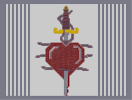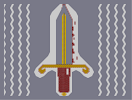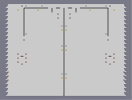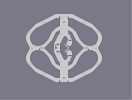True love Pointy dagger Lights, Camera, Action! Death Worm Line Tree C for Clarity

Pages: (0)

@ ghazbaran

The only thing I like about this one is the bike, which is quite choppy. The other stuff looked weird..

.

Lucky I didnt submit it without background, I'd get 1's as a gift

,

With this I learnt to paint background first

@seven_two
Why 2/5?

Hmm.

The last few guys pretty much nailed it. It's a great effort, but it's real rough around the edges. You've got those colours pretty much spot on. Try getting the shapes right.

I like the basic picture.

But yeah, it's far too rough around the edges, and other such little problems.

For effort.

3.5/5

The reason? It's complete. There are so many N-Arts (Including mine) that are not finished.

Why not any higher than a 3.5/5? real crude, the background has noticable white gaps, inside the bike there are white gaps, the tires on the bike are real crude, and general coloring is rough.